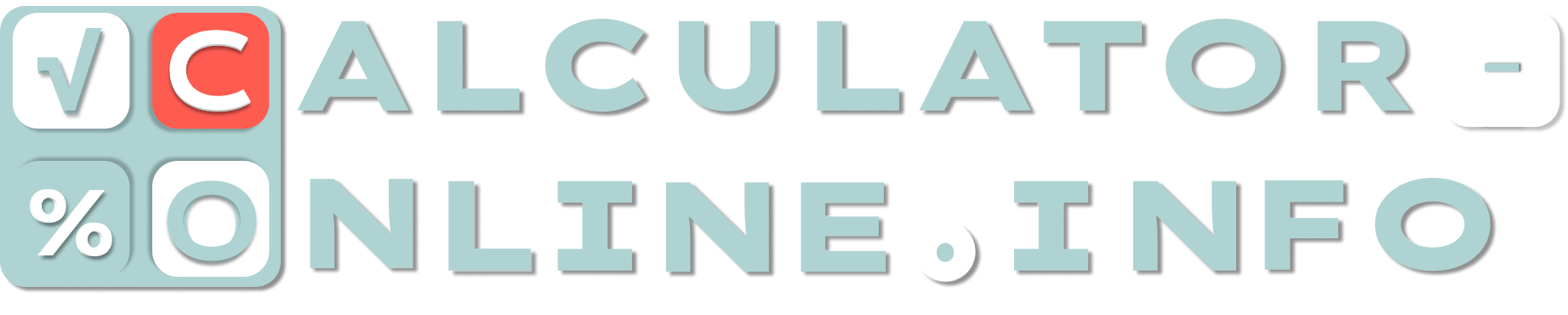# Price increase calculator | Calculate percentage change between old and new prices

What is the price change from final cost
\$
to initial cost
\$
=
0.00 %
×
Number of digits after the decimal point

Here is the price increase calculator which helps to calculate percent change between two costs. You can use it any quantity of times and with any numbers.

## How to calculate price increase percentage?

Price increase can be calculated with the help of this formula:

### Price change = (New price – Starting price) / Starting price × 100

This formula allows you to calculate price increase percentage. It means difference not in monetary terms, but in percentage terms. In case if new price is less than initial cost, you will be able to calculate price decrease.

### What price calculator does

Price percent calculator is digital tool that simplifies a calculation for users. It makes possible for you not to know the formula and count price change in one stage. Moreover, percentage price calculator is universal, so it can calculate both price increases and decreases.

### How to use price change calculator?

Since our tool is price increase percentage calculator and price decrease calculator at the same time, you need only to enter values of old price and current cost (after its increasing or decreasing). The result will appear automatically.

Rating
( 1 assessment, average 5 from 5 )Mathematician/ author of the article